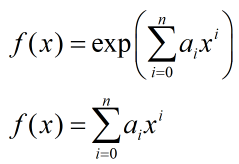2021-10-30 16:46

# 用指数多项式去拟合一组数据，用最小二乘法求解参数，如何加上0到40的积分为1这个约束``````x = [0:5:40]; % x随意
y = pi/80*sin(1/40*pi*x)+(rand(size(x))-0.5)*pi/80*1/2;% y随意设置，在正弦函数上加随机浮动
n = 5; % n随意
x = x(:);
y = y(:);
S = zeros(length(x), n+1);
q = ones(length(x),1);
S(:,n+1)=q;
for i = n:-1:1
q = q.*x;
S(:,i) = q;
end
A = S'*S;
b = S'*y;
a = A\b;%最小二乘
G = 1./(n+1:-1:1).*40.^(n+1:-1:1); %
aa = [A, G'; G, 0]\[b;1];%拉格朗日乘子法
``````
• 写回答
• 好问题 提建议
• 追加酬金
• 关注问题
• 邀请回答